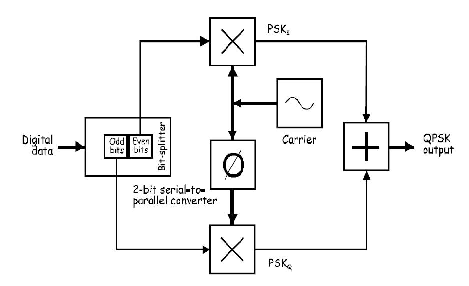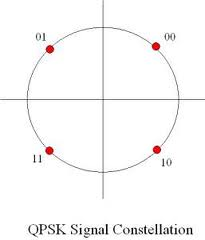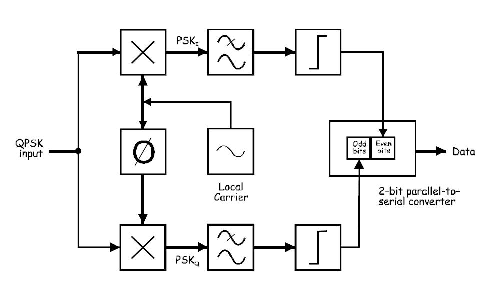. . . QPSK Modulation (Real time experiment) . . Generation of a QPSK signal: Figure below shows the block diagram of the mathematical implementation of QPSK.At the input of the modulator, the digital data’s even bits (i.e., bits 0,2,4 and so on) are stripped from the data stream by a “bit-splitter” and are multiplied with a carrier to generate a BPSK signal (called PSKI). At the same time, the data’s odd bits (i.e., bits 1,3,5 and so on) are stripped from the data stream and are multiplied with the same carrier to generate a second BPSK signal (called PSKQ). However, the PSKQ signal’s carrier is phase shifted by 90o before being modulated. The two BPSK signals are then simply added together for transmission and, as they have the same carrier frequency, they occupy the same portion of the radio frequency spectrum. While this suggests that the two sets of signals would be irretrievably mixed, the required 90o of phase separation between the carriers allows the sidebands to be separated by the receiver using phase discrimination. The constellation diagram of QPSK is as shown below:Each adjacent symbol only differs by one bit, sometimes known as quaternary or quadriphase PSK or 4-PSK, or 4-QAM. QPSK uses four points on the constellation diagram, equispaced around a circle. With four phases, QPSK can encode two bits per symbol shown in the diagram to minimize the BER- twice the rate of BPSK. Analysis shows that this may be used either to double the data rate compared to a BPSK system while maintaining the bandwidth of the signal or to maintain the data rate of BPSK but half the bandwidth needed. The implementation of QPSK is more general than that of BPSK and also indicates the implementation of higher order PSK.  Writing the symbols in the constellation diagram in terms of sine and cosine waves used to transmit them. This yields the four phases π/4, 3π/4, 5π/4 and 7π/4 as needed. This results in a two dimensional signal space with unit basis functions. The first basis function is used as the in-phase component of the signal and the second as the quadrature component of the signal. Hence the signal constellation consists of the signal- space 4 points.   Reception of a QPSK signal Figure below shows the block diagram of the mathematical implementation of QPSK demodulation.It is to be noticed that the arrangement uses two product detectors to simultaneously demodulate the two BPSK signals. This simultaneously recovers the pairs of bits in the original data. The two signals are cleaned-up using a comparator or some other signal conditioners then the bits are put back in order using a 2-bit parallel to serial converter. In order to understand how each detector picks out only one of the BPSK signals and not both of them, recall that the product detection of DSBSC signals is “phase sensitive”. That is, recovery of the message is optimal if the transmitted and local carriers are in phase with each another. But the recovered message is attenuated if the two carriers are not exactly in phase. Importantly, if the phase error is 90o, the amplitude of the recovered message is zero. It is to be noticed that the product detectors in the figure share the carrier but one of them is phase shifted 90o.That being the case, once the phase of the local carrier for one of the product detectors matches the phase of the transmission carrier for one of the BPSK signals, there is automatically a  90o phase error between the detector’s local carrier and the transmission carrier of the other BPSK signal. So the detector recovers the data on the BPSK signal that it’s matched to and rejects the other BPSK signal. Please enable JavaScript to view the comments powered by Disqus. Cite this Simulator:vlab.amrita.edu,. (2012). QPSK Modulation (Real time experiment). Retrieved 23 January 2022, from vlab.amrita.edu/?sub=59&brch=163&sim=1065&cnt=1 ..... ..... .....Copyright @ 2022 Under the NME ICT initiative of MHRD